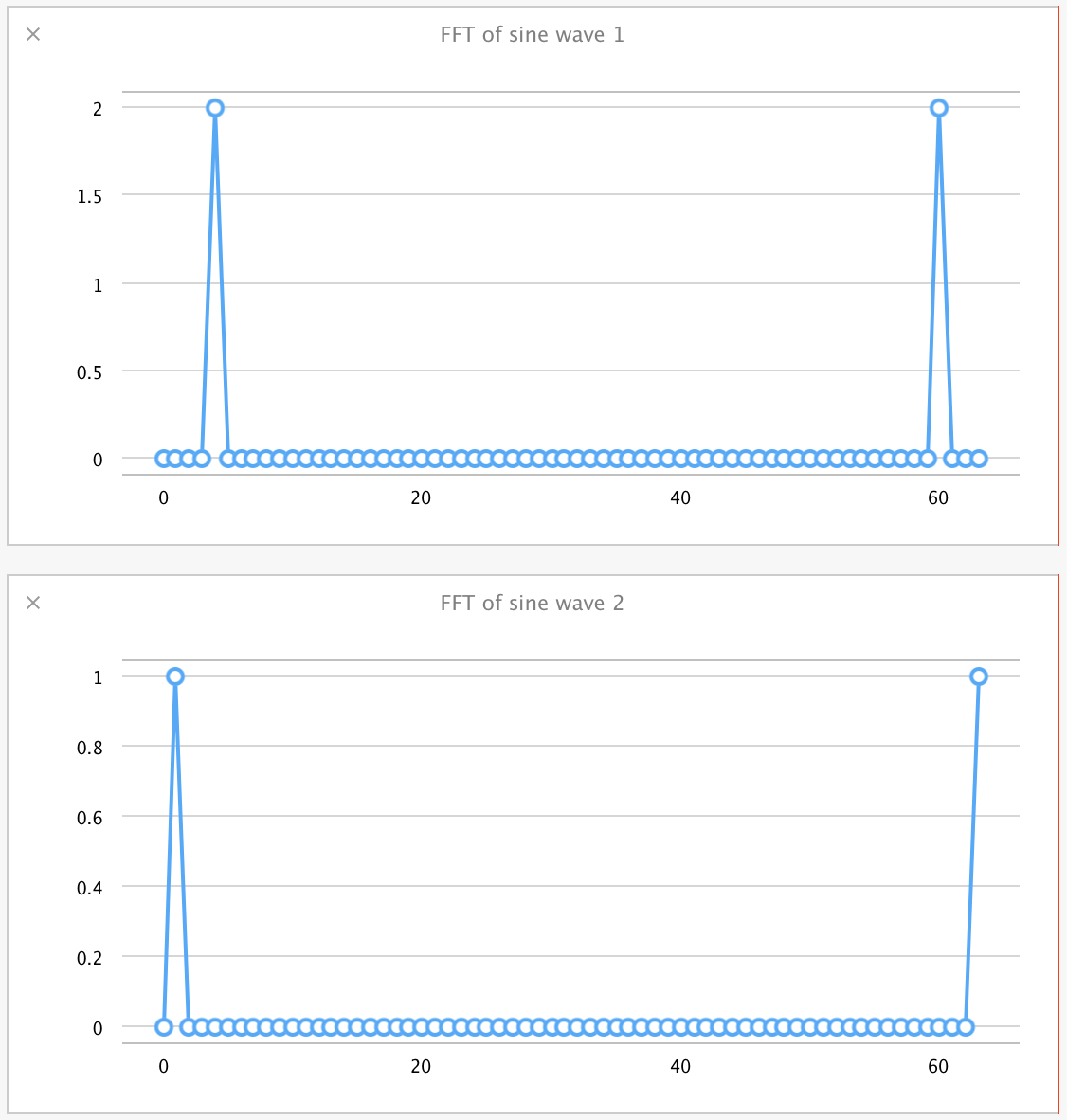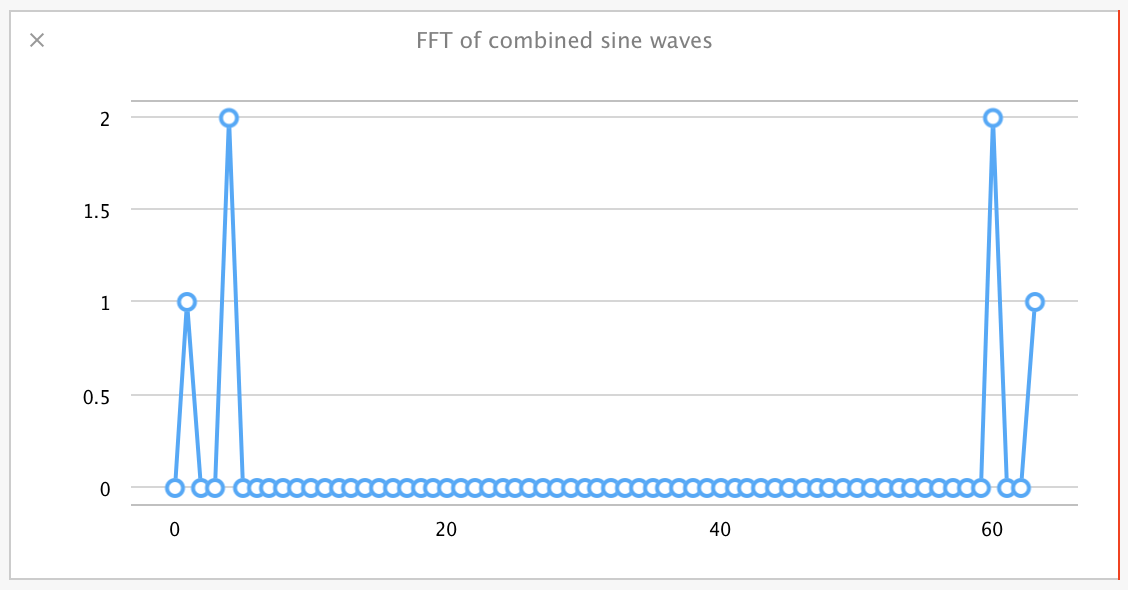Playground 快速原型制作

Swift 的 playground 主要还是作为学习这门语言的工具而被重视，然而我们只要关注一下类似项目，如 IPython notebooks，就能看到交互编程环境在更广阔的范围内的潜在应用。从科学研究机器视觉实验，这些任务现在都使用了 IPython notebooks。这种方式也被用来探索其他语言的范例，如 Haskell 的函数式编程

## 将 Playground 用于文档和测试

Swift 是一个全新的语言，许多人都使用 playground 来了解其语法和约定。不光是语言，Swift 还提供了一个新的标准库。目前这个标准库的文档中对于方法的说明不太详细，所以雨后春笋般的涌现了许多像 practicalswift.org 标准库方法列表这样的资源。

Playground 展示语法和实时执行真实数据的特性，为编写方法和库接口提供了很好的机会。为了介绍 Collection 方法的使用，我们创建了一个叫 CollectionOperations.playground 的例子，其中包含了一系列 collection 方法的例子，所有的样例数据都能实时修改。

``````let testArray = [0, 1, 2, 3, 4]
``````

``````let odds = testArray.filter{\$0 % 2 == 1}
``````

Playground 很大程度上会对我们如何执行测试驱动开发产生影响。Playground 能够快速展示我们所能做的东西，因此我们将比之前走得更快。但是同过去的测试驱动开发框架结合在一起时，能否走的更好？我们是否能提炼出更好的代码，以满足更少的缺陷数量和重构？

## 创建 Accelerate 的原型 -- 经过优化的信号处理

Accelerate 框架包括了许多功能强大的并行处理大型数据集的方法。这些方法可以利用例如 Intel 芯片中的 SSE 指令集，或者 ARM 芯片中的 NEON 技术等，这样的现代 CPU 中矢量处理指令的优势。然而，相较于功能的强大，它们的接口似乎有点不透明，其使用的文档也有点缺乏。这就导致许多开发者无法使用 Accelerate 这个强大的工具所带来的优势。

Swift 提供了一个机会，通过方法重载或为 Accelerate 框架进行包装后，可以让交互更加容易。这已经在 Chris Liscio 的库 SMUGMath 的实践中被证实，这也正是我们接下来将要创建的原型的灵感来源。

``````let sineArraySize = 64

let frequency1 = 4.0
let phase1 = 0.0
let amplitude1 = 2.0
let sineWave = (0..<sineArraySize).map {
amplitude1 * sin(2.0 * M_PI / Double(sineArraySize) * Double(\$0) * frequency1 + phase1)
}
``````

``````func plotArrayInPlayground<T>(arrayToPlot:Array<T>, title:String) {
for currentValue in arrayToPlot {
XCPCaptureValue(title, currentValue)
}
}
``````

``````plotArrayInPlayground(sineWave, "Sine wave 1")
``````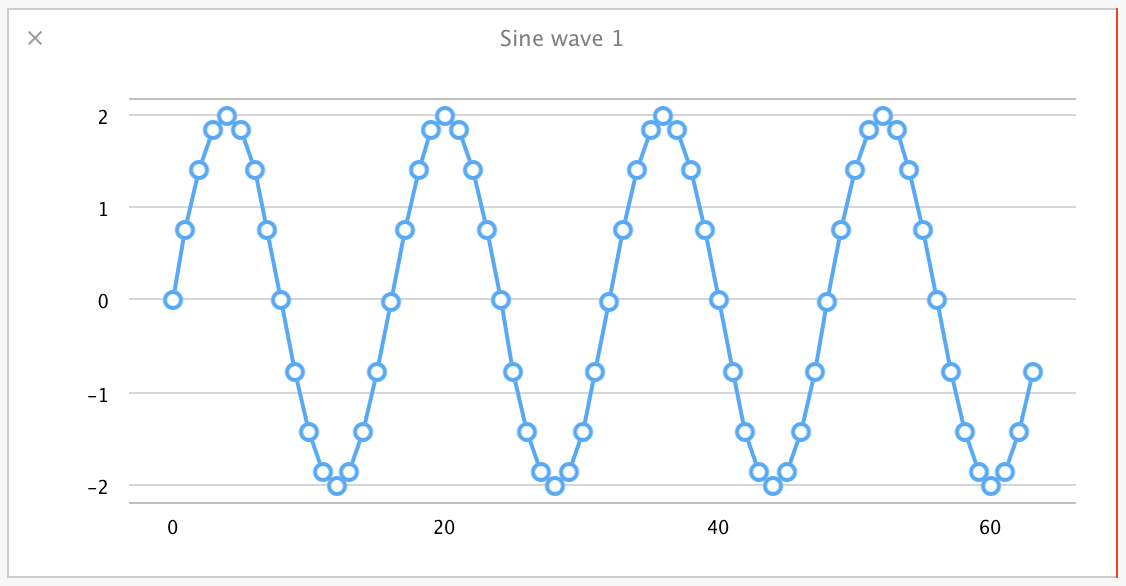``````let frequency2 = 1.0
let phase2 = M_PI / 2.0
let amplitude2 = 1.0
let sineWave2 = (0..<sineArraySize).map {
amplitude2 * sin(2.0 * M_PI / Double(sineArraySize) * Double(\$0) * frequency2 + phase2)
}
``````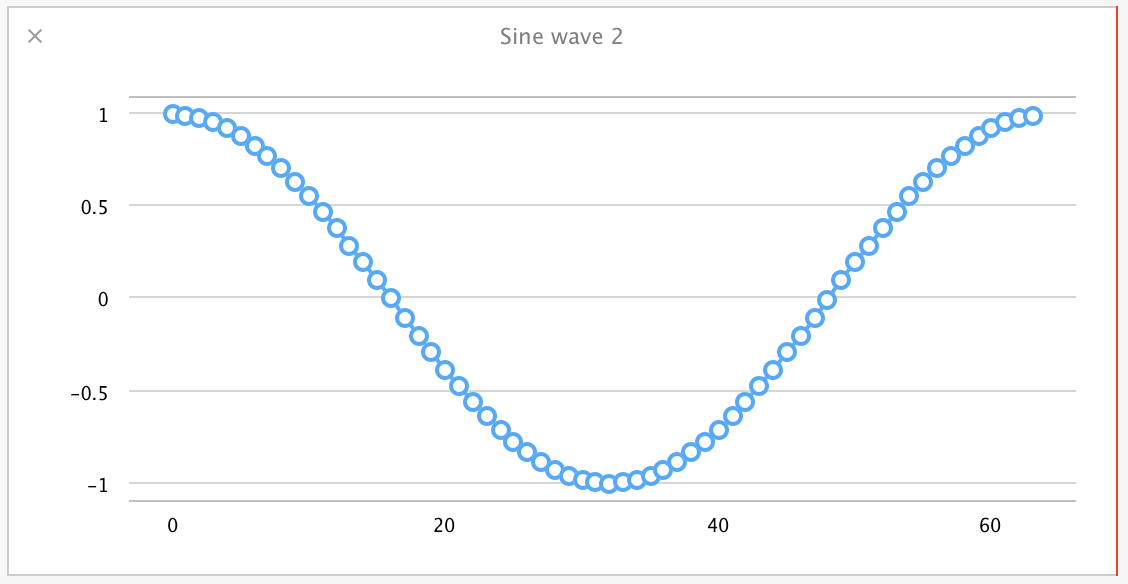``````infix operator  +++ {}
func +++ (a: [Double], b: [Double]) -> [Double] {
assert(a.count == b.count, "Expected arrays of the same length, instead got arrays of two different lengths")

var result = [Double](count:a.count, repeatedValue:0.0)
vDSP_vaddD(a, 1, b, 1, &result, 1, UInt(a.count))
return result
}
``````

``````var combinedSineWave = [Double](count:sineArraySize, repeatedValue:0.0)
for currentIndex in 0..<sineArraySize {
combinedSineWave[currentIndex] = sineWave[currentIndex] + sineWave2[currentIndex]
}

let combinedSineWave2 = sineWave +++ sineWave2

plotArrayInPlayground(combinedSineWave2, "Combined wave (Accelerate)")
``````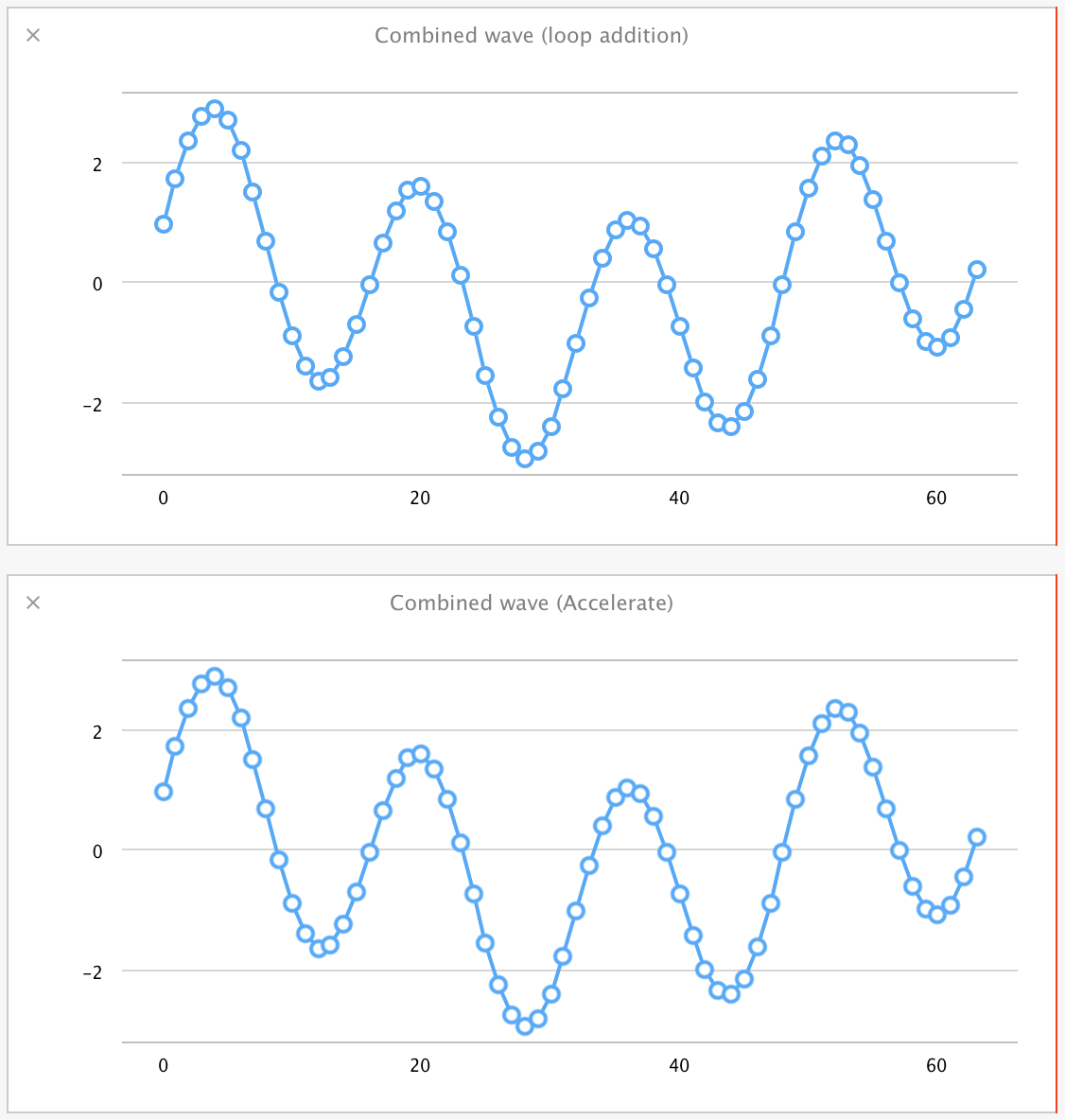Accelerate 的 vecLib 库中有很多等价的数学方法，包括平方根的 `vvsqrt()`。这是个使用方法重载的好例子，让我们来创建一个新版本的 `sqrt()`，使其能处理 `Double` 类型的数组。

``````func sqrt(x: [Double]) -> [Double] {
var results = [Double](count:x.count, repeatedValue:0.0)
vvsqrt(&results, x, [Int32(x.count)])
return results
}
``````

``````sqrt(4.0)
sqrt([4.0, 3.0, 16.0])
``````

``````let fft_weights: FFTSetupD = vDSP_create_fftsetupD(vDSP_Length(log2(Float(sineArraySize))), FFTRadix(kFFTRadix2))

func fft(var inputArray:[Double]) -> [Double] {
var fftMagnitudes = [Double](count:inputArray.count, repeatedValue:0.0)
var zeroArray = [Double](count:inputArray.count, repeatedValue:0.0)
var splitComplexInput = DSPDoubleSplitComplex(realp: &inputArray, imagp: &zeroArray)

vDSP_fft_zipD(fft_weights, &splitComplexInput, 1, vDSP_Length(log2(CDouble(inputArray.count))), FFTDirection(FFT_FORWARD));
vDSP_zvmagsD(&splitComplexInput, 1, &fftMagnitudes, 1, vDSP_Length(inputArray.count));

let roots = sqrt(fftMagnitudes) // vDSP_zvmagsD returns squares of the FFT magnitudes, so take the root here
var normalizedValues = [Double](count:inputArray.count, repeatedValue:0.0)

vDSP_vsmulD(roots, vDSP_Stride(1), [2.0 / Double(inputArray.count)], &normalizedValues, vDSP_Stride(1), vDSP_Length(inputArray.count))
return normalizedValues
}
``````